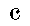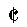Time Signatures

Time signatures tell you how many and what kind of notes per measure there are. The number on top is the number of notes per measure, and the bottom number is what kind of note. Let us explain further.

Let us take for example the most popular time signature, 4/4.
This means there is 4 quarter notes per measure. How is this so?

Looking at 4/4, you saw the 4 on top. You already knew that meant there were 4 somethings per measure. Then looking at the bottom number probably confused you. The bottom number can be 1, 2, 4, 8, 16, etc. Look at this chart.
 Bottom Number Value 1 Whole note 2 Half note 4 Quarter note 8 Eighth note 16 Sixteenth note

For example:
3/4 is 3 quarter notes per measure.
5/2 is 5 half notes per measure.
6/8 is 6 eighth notes per measure.

There are also 2 other common things you might see where the time signature should be.Common TimeSame as 4/4 timeCut TimeSame as 4/4 but everything is cut in half. Example: a half note = 1 quarter note, a whole note = 1 half note.

 Back to the... On to the... Clefs lesson Types of Notes

[ Introduction | Clefs | Time Signatures | Types of Notes | Types of Rests | Basic Counting I ]
[ Basic Counting II | Symbols | Shaping | Return to Education Section ]

Learning to Read Music, Created by Kevin Lux for